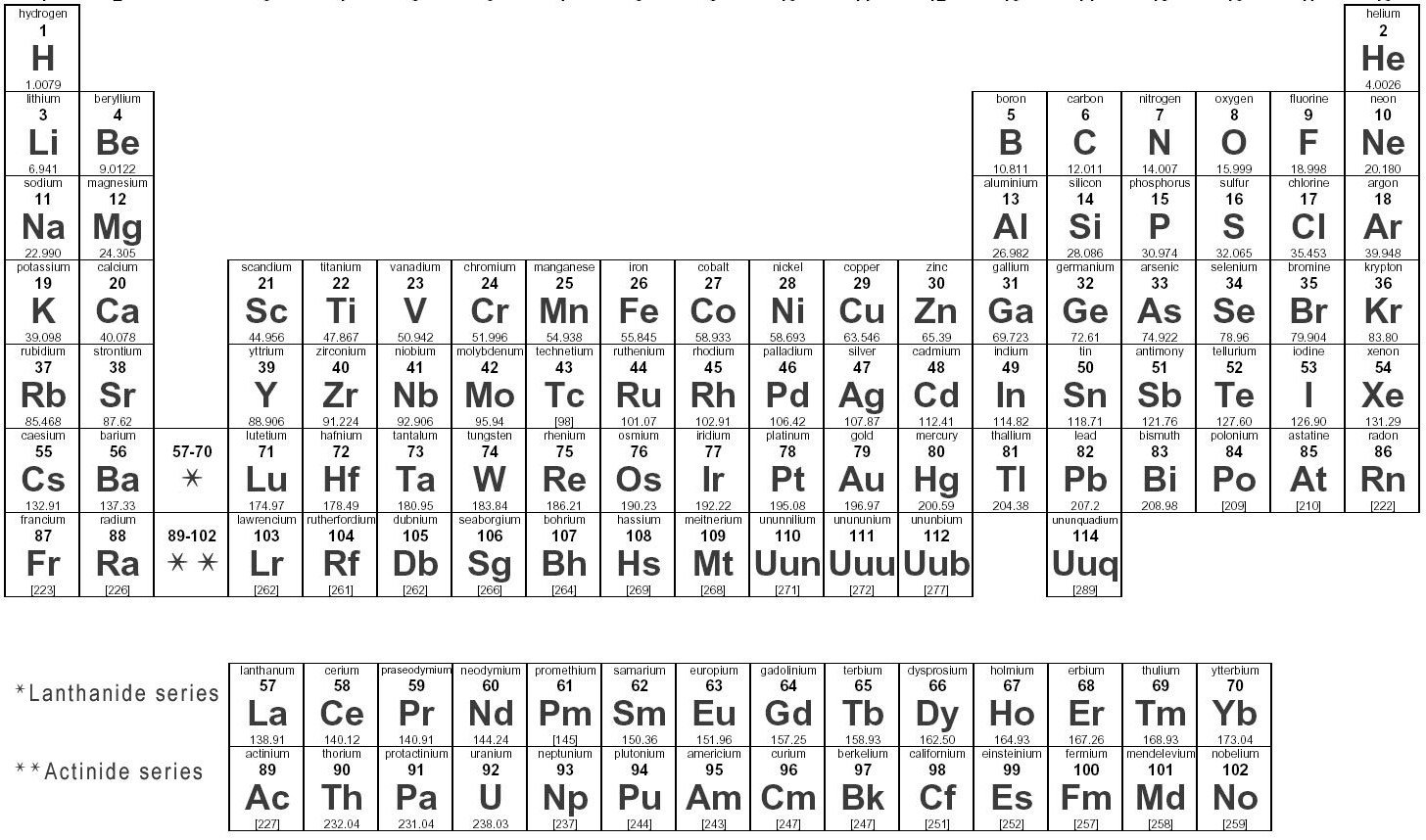Chemistry Semester 2 Final Exam
Show your work on scratch paper, especially when the problem says to!
Periodic Table of the ElementsLast Name *
First Name *
Period *
eMail
1. How many electrons does a neutral Carbon atom have? *
2. Magnesium commonly forms an ion with a charge of __________ *
3. What is the name of the family that contains Neon? *
4. Atomic Radius _____________ from left to right across a period and _____________ from top to bottom down a column on the periodic table. *
5. Which atom has the highest ionization energy, P, Mg, Mn, Cs? *
6. The last subshell/ending subshell electron configuration for Se is____________. You may use an AufBau Diagram. *
7. Which is the correct Noble Gas configuration for Aluminum? You may use an AufBau diagram. *
8. How many neutrons are in a neutral Bromine atom? *
9. What does Valence mean? *
10. In a Lewis Dot structure of Carbon TetraChloride how many dots would be drawn total? (Show drawing) *
11. In a Lewis Dot structure, how many dots will be drawn around a Carbon that is bonded to four other atoms? *
12. In a Lewis Dot structure of Carbon Dioxide, how many dots would be drawn in between the Carbon and one of the Oxygen atoms? (Show Drawing) *
13. Carbon Tetrachloride has a VSEPR geometry of ________ *
14. What type of intermolecular force involves a permanent polarity in a molecule? *
15. Which of these elements would form a polar bond with Carbon? (Show work) *
16. Electronegativity determines if a polar bond will form. Non-polar bonds form when _________ *
17. A molecule has a central atom with 3 atoms bonded to it and one lone pair of electrons. The shape will be _______________________ *
18. A tetrahedral molecule has a central Carbon surrounded by four Chlorine. Is this overall molecule polar? (Show drawing) *
19. In order for a substance to dissolve___________ *
20. Fill in the blanks ____Sodium Iodide + ___Chlorine gas ---> ____ Sodium Chloride + ____ Iodine gas (Show work) *
21. Using the equation from question 20, If you had 4 moles of Sodium Iodide how many moles of Iodine gas could you make? (Show work) *
22. Use the same equation from #20. How many grams of sodium chloride could you make with 0.29 grams of sodium iodide? (Show work) *
23. DiNitrogen Pentoxide plus Water yields two Nitric Acid ( N2O5 + H2O --> 2 HNO3 ). How many moles of water are needed to make 1.25 moles of Nitric Acid? (Show work) *
24. Using the same equation as question 23. How many grams of nitric acid will be produced when 0.25 moles of water are reacted? (Show work) *
25. What is the name of the process where you use given information to figure out the grams, moles or number of molecules in a chemical equation? *